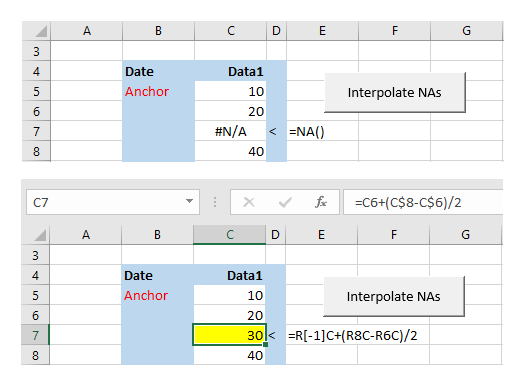LinearInterpolationFormulas

# Linear interpolation with FormulaR1C1

## Linear interpolation - example

The objective is to replace #N/A errors in a data vector (figure 1 top panel) with formulas returning the interpolated value (figure 1 bottom panel).Fig 1: Interpolation example - sample NA vector in column C (top panel), and interpolated value, with A1 style in FormulaBar and R1C1 returned by the Excel FORMULATEXT function (bottom panel)

The VBA code is provided in the next section.

## Linear interpolation - the VBA code

Code 1: Macro InterpFormulas replaces NA errors with interpolation formulas
Sub InterpFormulas()
' Type declaration characters (TDC): % Integer; & Long
Dim Top&, Bot&
Dim Run As Double
Dim i%, j%, k%
Dim DataPoints&, RefRow&, LastRow&
Dim Ref As Range
Dim Wsf As WorksheetFunction

Set Wsf = WorksheetFunction
Set Ref = Range("Anchor").Offset(0, 1)

' Coordinates
LastRow = Ref.End(xlDown).Row
RefRow = Ref.Row    ' Anchor row
DataPoints = LastRow - RefRow

' Identify NA block
For i = 0 To DataPoints
If IsNumeric(Ref.Offset(i, 0)) And Wsf.IsNA(Ref.Offset(i + 1, 0)) Then
Top = Ref.Offset(i, 0).Row
j = i
ElseIf IsNumeric(Ref.Offset(i + 1, 0)) And Wsf.IsNA(Ref.Offset(i, 0)) Then
Bot = Ref.Offset(i, 0).Row + 1
Run = Bot - Top

' Write formulas
For k = 1 To Run - 1
Ref.Offset(k + j, 0).FormulaR1C1 = "=R[-1]C+(R" & Bot & "C-R" & Top & "C)/" & Run
Ref.Offset(k + j, 0).Interior.ColorIndex = 6
Next k
End If
Next i

End Sub


• NA block (coordinate) is the series of consecutive NA errors and the adjacent top and bottom numeric values. Rows 6 to 8 in figure 1
• Line 21: Top (coordinate) is the top numeric value with NA offset +1 row. Cell C6 with value 20 in figure 1
• Line 24: Bottom (coordinate) is the (bot)tom numeric value with NA offset -1 row. Cell C8 with value 40 in figure 1
• Using row 7 (cell C7) as an example, the values of Top, Bot, and Run are (figure 2):Fig 2: Watches Window - interpolation variables Top, Bot, and Run
• Line 29: FormulaR1C1 "=R[-1]C+(R" & Bot & "C-R" & Top & "C)/" & Run, returns
"=R[-1]C+(R8C-R6C)/2", equivalent to
=C6+(C$8-C$6)/2
• Line 30 adds the Yellow Fill (VBA Interior property) to each cell containing the interpolation formula

## The Reset procedure

Code 2 resets the Data1 range (Range("Anchor").Offset(0, 1)) by copying the replacement data from the TestData range. The Paste argument (line 46) overwrites the Yellow Fill color from code 1 line 30.

Code 2: Macro ResetData overwrites the interpolated values and clears the yellow format
Sub ResetData()